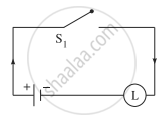# Obtain the simple logical expression of the following. Draw the corresponding switching circuit. p ∨ (q ∧ ∼ q) - Mathematics and Statistics

Sum

Obtain the simple logical expression of the following. Draw the corresponding switching circuit.

p ∨ (q ∧ ∼ q)

#### Solution

Using the laws of logic, we have,
p ∨ (q ∧ ∼ q)
≡ p ∨ F .......(By Complement Law)
≡ p ...........(By Identity Law)
Hence, the simple logical expression of the given expression is p.
Let p: the switch S1 is closed
Then the corresponding switching circuit is:Concept: Application of Logic to Switching Circuits
Is there an error in this question or solution?